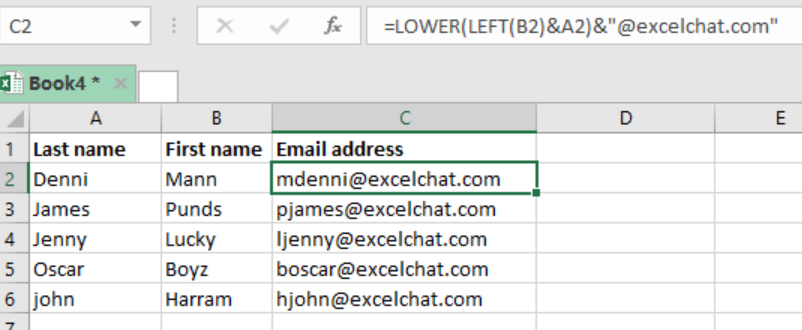Get instant live expert help with Excel or Google Sheets“My Excelchat expert helped me in less than 20 minutes, saving me what would have been 5 hours of work!”

#### Post your problem and you’ll get Expert help in seconds.

Your message must be at least 40 characters
Our professional Expert are available now. Your privacy is guaranteed.

# Create email address from nameRead time: 20 minutes

There are times when we are required to create email address from name when working with excel spreadsheets. This article provides a quick guide on the process.Figure 1: Example of how to create email address from name

## General syntax of the formula

`=LOWER(LEFT(first) & last) “@domain.com”`

## Understanding the formula

It is actually easy to build an email address using the first and last name. What you will be required to do is to use a formula that concatenates values, with the help of LOWR and LEFT functions.

## How the formula works

• If we want to build an email address from the name “Jenny Lucky” the formula will bring ljenny@excelchat.com.
• In the formula, the FIRST function is fundamental as it helps us get the first letter of the first name. in our case, this is in column B.
• The LEFT function will then get the “num_chars” value for the second argument. If the second argument is left blank, the default one will be 1. Note that this argument is optional.
• By the help of the concatenation operator (&), the letter from the first name is joined to the last name.
• The result of this is then wrapped by the LOWER function. This function will force all the text in it to lowercase.
• We then join the result to “@”, then domain to get the email address we are looking for.

## Instant Connection to an Expert through our Excelchat Service

Most of the time, the problem you will need to solve will be more complex than a simple application of a formula or function. If you want to save hours of research and frustration, try our live Excelchat service! Our Excel Experts are available 24/7 to answer any Excel question you may have. We guarantee a connection within 30 seconds and a customized solution within 20 minutes.

### Did this post not answer your question? Get a solution from connecting with the expert.Another blog reader asked this question today on Excelchat:
Solution examplesI need to create a formula that extracts the prefix from column A
Solved by I. Q. in 42 minsi need a formula to combine column y with the number mentioned in column w counting in from 1 to 0 and posting the total count next to number 1 in column v.
Solved by E. J. in 60 minsI would like to insert a formula to add something (Maybe a 'Y') if a cell contains 14 days or less. Is this possible?
Solved by F. H. in 6 minsWhat is the formula for splitting up one column text into 2 columns?
Solved by B. H. in 20 minsTrying to write a formula to pull all values from a cell that are LEFT of a hyphen "-", or all values if no hyphen
Solved by F. L. in 54 mins## Subscribe to Excelchat.coAnother blog reader asked this question today on Excelchat: